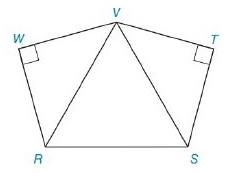Chapter 8.2, Problem 30EElementary Geometry For College St...

7th Edition
Alexander + 2 others
ISBN: 9781337614085

Solutions

Chapter
SectionElementary Geometry For College St...

7th Edition
Alexander + 2 others
ISBN: 9781337614085
Textbook Problem

In Exercises 27 to 30, find the area of the figure shown. Given: Pentagon R S T V W with m ∠ V R S = m ∠ V S R = 60 ° ,   R S = 8 2 , and R W - ≅ W V - ≅ V T - ≅ T S - Find: A R S T V WTo determine

To Find:

Area of the pentagon provided.

Explanation

Formula Used:

1. Split the pentagon into three triangles and find the corresponding areas and then sum it up.

2. Pythagorean theorem for the right angle triangle ABC for the hypotenuse AC,

AC2=AB2+BC2.

3. Sum of the angles in a triangle is 180°.

4. Area of right triangle =12bh, where b is the base and h is the height of the triangle.

5. Area of the equilateral triangle ABC with each side of length s is given by AABC= S243.

Let the vertices of the provided pentagon be R,S,T,V and W.

Let’s divide the pentagon into three triangles. That is, RVW,  SVT and RVS.

It is given that VSR=mVSR=60°, RS=82, and RW-WV-VT-TS-.

Now consider the triangle RVS,

Here mVRS=mVSR=60° and RS=82.

mVRS+mVSR+ mRVS=180°

mRVS=180°-120°=60°

Therefore, RVS is an equilateral triangle.

Area of the equilateral triangle = S243, s is the side lengths

Still sussing out bartleby?

Check out a sample textbook solution.

See a sample solution

The Solution to Your Study Problems

Bartleby provides explanations to thousands of textbook problems written by our experts, many with advanced degrees!

Get Started

Find the slope of the line through P and Q. 10. P(1, 4), Q(6, 0)

Single Variable Calculus: Early Transcendentals, Volume I

Evaluate the integrals in Problems 1-32. 13.

Mathematical Applications for the Management, Life, and Social Sciences

Finding a Limit In Exercises 87-94, find limx0f(x+x)f(x)x f(x)=1x+3

Calculus: Early Transcendental Functions (MindTap Course List)

Sometimes, Always, or Never: (ax) = ax.

Study Guide for Stewart's Single Variable Calculus: Early Transcendentals, 8th

For 0

Study Guide for Stewart's Multivariable Calculus, 8th